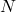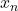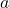# Computing Log-Sum-Exp

This post is about a computational trick that everyone should know, but that doesn’t tend to be explicitly taught in machine learning courses.  Imagine that we have a set ofvalues,and we want to compute the quantity

(1)This comes up all the time when you want to parameterize a multinomial distribution using a softmax, e.g., when doing logistic regression and you have more than two unordered categories.  If you want to compute the log likelihood, you’ll find such an expression due to the normalization constant.  Computing this naively can be a recipe for disaster, due to underflow or overflow, depending on the scale of the.  Consider a simple example, with the vector.  This seems pretty straightforward, and we get about.  Now what about.  This seems like it should also be straightforward, but instead our computer gives us back.  If we trywe get.  What’s happening here?  Well, in your typical 64-bit double,anddue to overflow and underflow, respectively.  Even though the log would make the numbers reasonably scaled again with infinite precision, it doesn’t work on a real computer with typical floating point operations.  What to do?

The trick to resolve this issue is pretty simple and exploits the identity:

(2)for any.  This means that you are free to shift the center of the exponentiated variates up and down, however you like.  A typical thing to do is to make

(3)which ensures that the largest value you exponentiate will be zero.  This means you will definitely not overflow and even if the rest underflow you will still get a reasonable value.

## 2 Comments on “Computing Log-Sum-Exp”

1.Interesting. You know, I’ve long been doing this for marginalizing posterior probabilities. Like, log p(theta|x) = log sum_i[p(theta|x, yi)p(y_i)]. Usually I have the logarithm of all the p(theta|x, yi), and I add or subtract from each of them as convenient because the normalizing constant is unknown anyway.

But it actually never occurred to me that I could be using this same trick even for numbers that I know exactly, by just adding back in whatever factor I removed. (by “factor” I mean here each term is divided by exp(a) and in the end the sum is effectively multiplied by exp(a), so the “factor” I’m talking about is exp(a))